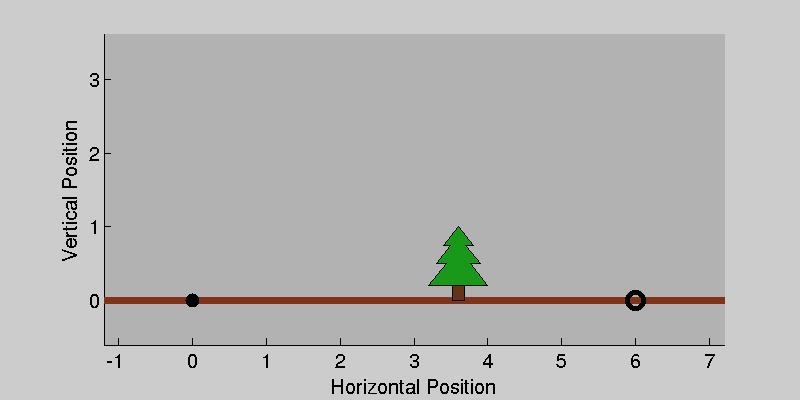# Cannon Example

- Matthew Kelly

Suppose that you have a cannon, and you would like to hit a known target. To make things interesting, let's assume that there is air friction and that you would like to minimize the amount of powder used for the shot.

This situation can be modeled as a boundary-value-problem, since you know where the trajectory (path of the cannon ball) should start and end. The goal is to find the trajectory, including the initial velocity of the cannon ball. We will assume that:
(1) the cannon ball is a point mass,
(2) air friction is modeled using quadratic drag, and
(3) amount of powder is proportional to initial speed squared.
Thus we can write out the problem and draw a nice picture as shown below.## Solution: Single Shooting

Single shooting works by approximating the trajectory using a single simulation. In other words, fire the cannon, check where the ball landed, adjust the initial speed, repeat. In math terms, the error between the target and where the cannon ball landed is called a defect. The optimization algorithm will try to find the initial speed such that this defect driven to zero. It turns out that single shooting is an effective method for simple problems (like this one), but it will fail on problems that are more difficult.## Solution: Multiple Shooting

Multiple shooting is very similar to single shooting, but it turns out to be much more robust on difficult problems. It works by breaking the original problem into many small problems, and then solving them in parallel. At the end, each segment is constrained to connect to the previous one.## Solution: Direct Collocation

Direct collocation is a bit more difficult to visualize, since there is no simulation step. Instead, the trajectory is approximated using a piecewise polynomial. Physics are satisfied by requiring that the dynamics (derivative of the state) match the derivative of the polynomial at each collocation point - the points that implicitly define the polynomial. In effect, shooting methods satisfy dynamics using explicit integration, while collocation methods use implicit integration.

There are a wide variety of collocation schemes. Orthogonal collocation schemes, developed for computing satellite trajectories, use a small number of high-order polynomials. They are extremely accurate for problems well behaved solutions. The other extreme is direct collocation, which uses a large number of low-order polynomials to represent the solution. It tends to be slightly less accurate, but can be more robust. Some sophisticated methods (GPOPS) use an adaptive meshing routine to iterative change the number of segments and order of the polynomials.

I gave a presentation at the Cornell robotics seminar on direct collocation in December 2015. You can view the presentation as a web-page here.